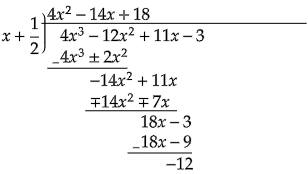# Using remainder theorem, find the remainder when $f( x)$ is divided by $g( x)$:$f( x)=4 x^{3}-12 x^{2}+11 x-3,\ g( x)=x+\frac{1}{2}$.

Given: $f( x)=4 x^{3}-12 x^{2}+11 x-3$ and $g( x)=x+\frac{1}{2}$.

To do: To find the remainder when $f( x)$ is divided by $g( x)$.

Solution:

As given, $f( x)=4 x^{3}-12 x^{2}+11 x-3$ and $g( x)=x+\frac{1}{2}$.

On dividing $f( x)$ by $g( x)$:Thus, when we divide $f( x)$ by $g( x)$, the remainder is $-12$.

Updated on: 10-Oct-2022

45 Views